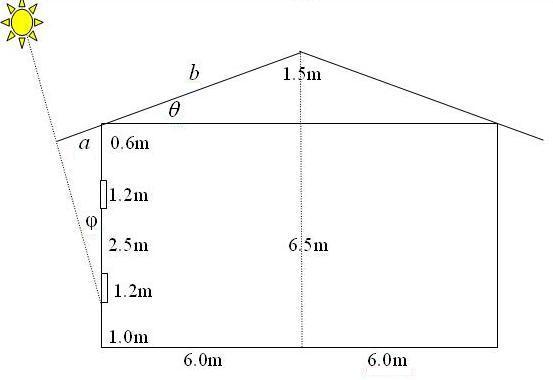#### You may also likeFour rods are hinged at their ends to form a convex quadrilateral. Investigate the different shapes that the quadrilateral can take. Be patient this problem may be slow to load.Given a square ABCD of sides 10 cm, and using the corners as centres, construct four quadrants with radius 10 cm each inside the square. The four arcs intersect at P, Q, R and S. Find the area enclosed by PQRS.### Get Cross

A white cross is placed symmetrically in a red disc with the central square of side length sqrt 2 and the arms of the cross of length 1 unit. What is the area of the disc still showing?

# Raising the Roof

##### Age 14 to 16Challenge LevelThe diagram shows a cross-sectional view of a house. The roof space is 12 metres across and 1.5 metres high at the highest point in the centre. Find the angle of slope of the roof.
Also find the sloping distance $b$ from the top of the roof to the top of the walls.

The hottest part of the day is when the sun is directly overhead. The roof overhangs by a distance $a$ and it shades all the windows from the sun while the sun's rays are inclined at 8 degrees or less to the vertical. The lowest part of the windows is one metre above the ground and the angle $\phi$ in the diagram is 8 degrees. Find the length of the overhang, $a$.